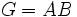# Every element of a finite field is expressible as a sum of two squares

(diff) ← Older revision | Latest revision (diff) | Newer revision → (diff)

## History

This result is attributed to Henry Mann.

## Statement

Suppose$k$ is a finite field. Then, every element$x \in k$ can be expressed in the form$x = a^2 + b^2$, where$a,b \in k$.

## Facts used

1. Multiplicative group of a finite field is cyclic: We actually only need the weaker statement that, for a field of odd characteristic, exactly half the elements of the multiplicative group are squares.
2. Product of subsets whose total size exceeds size of group equals whole group: If$A,B$ are subsets of a finite group$G$, where$|A| + |B| > |G|$, then$G = AB$.

## Proof

### Case of characteristic two

In this case, the square map is surjective and every element is a square, because the multiplicative group is of odd order.

### Case of odd characteristic

1. Reasoning in the multiplicative group: Suppose$k$ has$q$ elements. Then its multiplicative group$k^\times$ has$q - 1$ elements. By fact (1), the multiplicative group is cyclic of order$q - 1$, which is even. Thus, exactly half the elements (corresponding to even powers of the generator) are squares. Since$0$ is also a square, we obtain$(q + 1)/2$ elements of$k$ that are squares.
2. Reasoning in the additive group: We now apply fact (2) with$G$ as the additive group of$k$, which has size$q$, and both$A$ and$B$ as equal to the set of (multiplicative) squares, which has size$(q + 1)/2$.